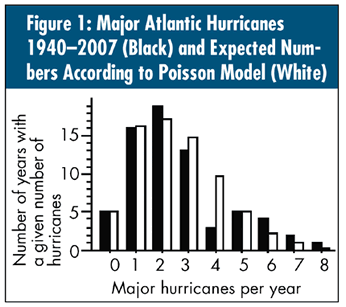# Quality DigestBio

## Probability Models Don’t Generate Your Data

### Don’t start your analysis by asking, “How are these data distributed?”

The number of major hurricanes in the Atlantic since 1940 (as we considered in my February column, “First, Look at the Data”) are shown as a histogram in figure 1, below. Some analysts would begin their treatment of these data by considering whether they might be distributed according to a Poisson distribution.

The 68 data in figure 1 have an average of 2.60. Using this value as the mean value for a Poisson distribution, we can carry out any one of several tests collectively known as “goodness-of-fit” tests. Skipping over the details, the results show that there’s no detectable lack of fit between the data and a Poisson distribution with a mean of 2.60. Based on this, many analysts would proceed to use techniques that are appropriate for collecting Poisson observations. For example, they might transform the data in some manner, or they might compute probability limits to use in analyzing these data. Such actions would be wrong on several levels.

First of all, because the analysis in figure 1 began with a goodness-of-fit test, we must understand just what that test does and doesn’t do. The key is in the language used. The statement, “There’s no detectable lack of fit” isn’t the same as saying, “These data were generated using a particular probability model.” The double negative isn’t the same as the positive. However, too often in practice, the double negative is used as if it were a positive statement. In fact, with enough data, you’ll always reject any particular probability model. This is why all goodness-of-fit tests are actually lack-of-fit tests, and using the correct terminology helps to prevent this error.

The only time we can ever make a positive statement that the data were generated using a particular probability model is when generating artificial data sets. Although this may be appropriate when working out the details for a new type of analysis, it has no place in analyzing real-world data.

Don’t we need to know if the data are normally distributed, or if they satisfy certain conditions before we can use our analysis techniques? No. This notion comes from confusing the purpose of data analysis and the way we teach statistical inference. When we analyze data, we want to know if a change has occurred, or if two things are different, because we’re going to take action based on the outcome of the analysis. As long as we are reasonably sure about our decision, the particular alpha level, or confidence level, is no longer important. As long as our procedure is reasonably conservative, so that the potential signals aren’t readily confused with the probable noise, we have a technique we can use.

In statistical inference, we rarely apply distributional assumptions to the original data, X. Instead, we work with some transformation of the data, Y. A statistic Y will generally have a known distribution, which will characterize some aspect of X’s distribution. Using Y’s known distribution, we compute critical values, or P-values, and then decide what this tells us about the distribution of X.

Thus, a common point of confusion for statistics students is thinking the distribution of X must be known before performing any analysis. Thankfully this isn’t true. In fact, this point of confusion was a major obstacle to developing analytical techniques during the 1800s.

Finally, the overwhelming obstacle to using the question of how the data are distributed as the starting point for an analysis is the fact that the data might not be homogeneous. In February’s column, the data in figure 1 came from two different systems. For 33 years the number of major hurricanes averaged about 1.7 per year, with an upper bound of four per year. For the other 35 years, the multidecadal tropical oscillation averaged 3.5 major hurricanes per year, with an upper bound of nine or 10 per year. So which of these two different systems does the Poisson model in figure 1 represent? Neither.Your data are the result of a process or system, and like all things in this world, these processes and systems are subject to change. For this reason alone, the primary question of data analysis is, and always has been, “Are these data reasonably homogeneous, or do they contain evidence of a lack of homogeneity?” The primary tool for examining any set of data for homogeneity is the process-behavior chart. This is why any data analysis should always begin by using the context to chart the data in a rational manner. To do otherwise may well result in patent nonsense.

### Discuss